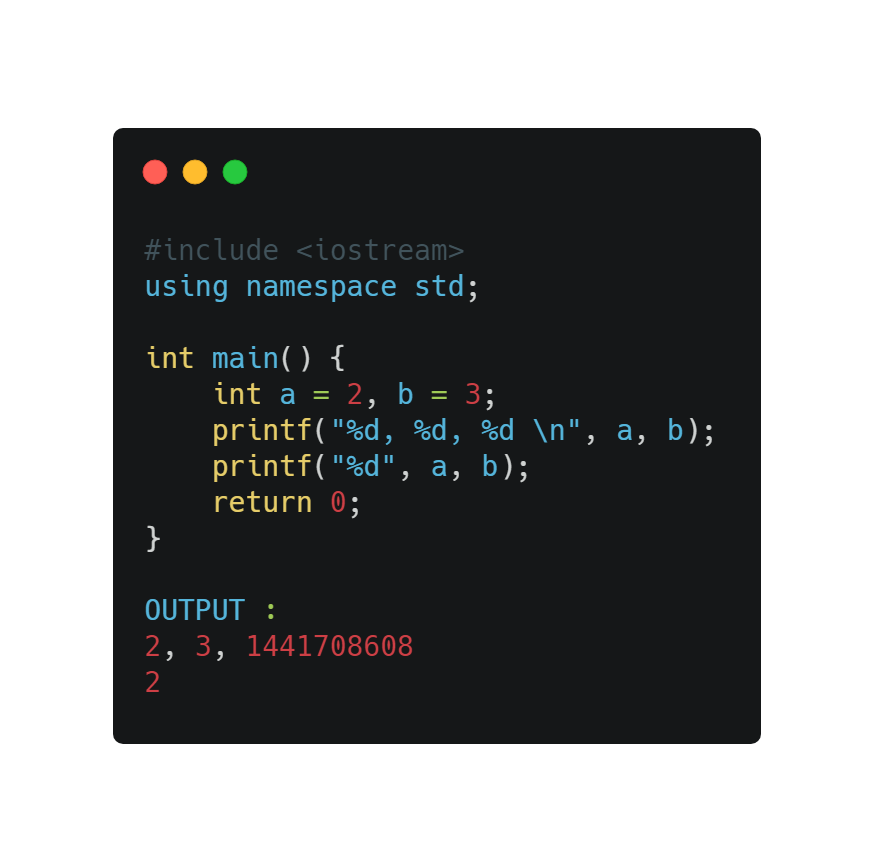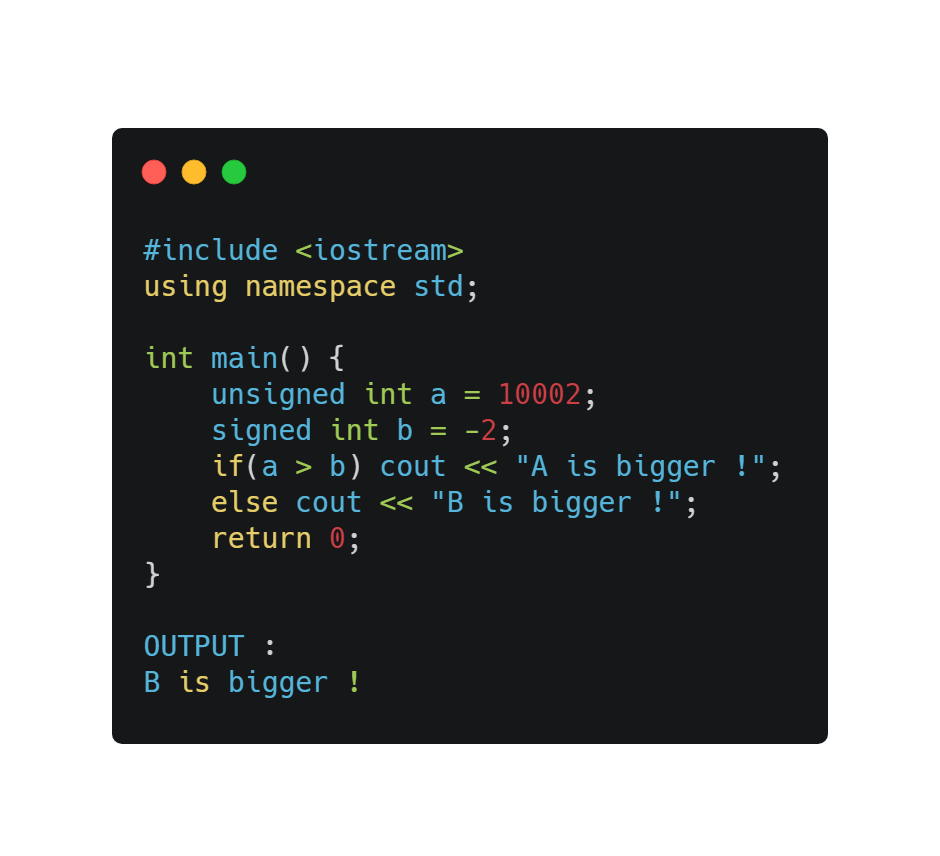1649887200

# TinySpline: ANSI C Library for NURBS, B-Splines and Bézier, Curves

## TinySpline

TinySpline is a small, yet powerful library for interpolating, transforming, and querying arbitrary NURBS, B-Splines, and Bézier curves. The core of the library is written in ANSI C (C89) with a C++ wrapper for an object-oriented programming model. Based on the C++ wrapper, auto-generated bindings for C#, D, Go, Java, Javascript, Lua, Octave, PHP, Python, R, and Ruby are provided.

### Features

• Object-oriented programming model
• B-Splines of any degree and dimensionality
• Spline interpolation
• Cubic natural
• Centripetal Catmull–Rom
• Evaluation
• Knots
• Sampling (multiple knots at once)
• Components (find y for given x)
• Knot insertion (refinement)
• Bézier curve decomposition
• Derivative
• Degree elevation
• Computation of rotation minimizing frames
• Morphing
• Serialization (JSON)
• Vector math

### Installation

#### Pre-built Binaries

Conan (C/C++):
https://conan.io/center/tinyspline

NuGet (C#):

``````<PackageReference Include="tinyspline" version="0.4.0.1" />
``````

Go:

``````go get github.com/tinyspline/go@v0.4.0
``````

Luarocks (Lua):

``````luarocks install --server=https://tinyspline.github.io/lua tinyspline
``````

Maven (Java):

``````<dependency>
<groupId>org.tinyspline</groupId>
<artifactId>tinyspline</artifactId>
<version>0.4.0-1</version>
</dependency>
``````

PyPI (Python):

``````python -m pip install tinyspline
``````

On macOS, you may need to change the path to Python in `_tinysplinepython.so` via `install_name_tool`.

See BUILD.md.

### Getting Started

A variety of examples (unit tests) can be found in the test subdirectory. The examples subdirectory contains at least one example for each interface (target language).

The following listing shows a python example:

``````from tinyspline import *
import matplotlib.pyplot as plt

spline = BSpline.interpolate_cubic_natural(
[
100, -100, # P1
-100,  200, # P2
100,  400, # P3
400,  300, # P4
700,  500  # P5
], 2) # <- dimensionality of the points

# Draw spline as polyline.
points = spline.sample(100)
x = points[0::2]
y = points[1::2]
plt.plot(x, y)

# Draw point at knot 0.3.
vec2 = spline.eval(0.3).result_vec2()
plt.plot(vec2.x, vec2.y, 'ro')

# Draw tangent at knot 0.7.
pos = spline(0.7).result_vec2() # operator () -> eval
der = spline.derive()(0.7).result_vec2().norm() * 200
s = (pos - der)
t = (pos + der)
plt.plot([s.x, t.x], [s.y, t.y])

plt.show()
``````

The resulting image:### Theoretical Backgrounds

 is a very good starting point for B-Splines.

 explains De Boor's Algorithm and gives some pseudo code.

 provides a good overview of NURBS with some mathematical background.

 is useful if you want to use NURBS in TinySpline.

Author: msteinbeck
Source code: https://github.com/msteinbeck/tinyspline

## Buddha Community1649887200

## TinySpline

TinySpline is a small, yet powerful library for interpolating, transforming, and querying arbitrary NURBS, B-Splines, and Bézier curves. The core of the library is written in ANSI C (C89) with a C++ wrapper for an object-oriented programming model. Based on the C++ wrapper, auto-generated bindings for C#, D, Go, Java, Javascript, Lua, Octave, PHP, Python, R, and Ruby are provided.

### Features

• Object-oriented programming model
• B-Splines of any degree and dimensionality
• Spline interpolation
• Cubic natural
• Centripetal Catmull–Rom
• Evaluation
• Knots
• Sampling (multiple knots at once)
• Components (find y for given x)
• Knot insertion (refinement)
• Bézier curve decomposition
• Derivative
• Degree elevation
• Computation of rotation minimizing frames
• Morphing
• Serialization (JSON)
• Vector math

### Installation

#### Pre-built Binaries

Conan (C/C++):
https://conan.io/center/tinyspline

NuGet (C#):

``````<PackageReference Include="tinyspline" version="0.4.0.1" />
``````

Go:

``````go get github.com/tinyspline/go@v0.4.0
``````

Luarocks (Lua):

``````luarocks install --server=https://tinyspline.github.io/lua tinyspline
``````

Maven (Java):

``````<dependency>
<groupId>org.tinyspline</groupId>
<artifactId>tinyspline</artifactId>
<version>0.4.0-1</version>
</dependency>
``````

PyPI (Python):

``````python -m pip install tinyspline
``````

On macOS, you may need to change the path to Python in `_tinysplinepython.so` via `install_name_tool`.

See BUILD.md.

### Getting Started

A variety of examples (unit tests) can be found in the test subdirectory. The examples subdirectory contains at least one example for each interface (target language).

The following listing shows a python example:

``````from tinyspline import *
import matplotlib.pyplot as plt

spline = BSpline.interpolate_cubic_natural(
[
100, -100, # P1
-100,  200, # P2
100,  400, # P3
400,  300, # P4
700,  500  # P5
], 2) # <- dimensionality of the points

# Draw spline as polyline.
points = spline.sample(100)
x = points[0::2]
y = points[1::2]
plt.plot(x, y)

# Draw point at knot 0.3.
vec2 = spline.eval(0.3).result_vec2()
plt.plot(vec2.x, vec2.y, 'ro')

# Draw tangent at knot 0.7.
pos = spline(0.7).result_vec2() # operator () -> eval
der = spline.derive()(0.7).result_vec2().norm() * 200
s = (pos - der)
t = (pos + der)
plt.plot([s.x, t.x], [s.y, t.y])

plt.show()
``````

The resulting image:### Theoretical Backgrounds

 is a very good starting point for B-Splines.

 explains De Boor's Algorithm and gives some pseudo code.

 provides a good overview of NURBS with some mathematical background.

 is useful if you want to use NURBS in TinySpline.

Author: msteinbeck
Source code: https://github.com/msteinbeck/tinyspline1590609660

## Count of triplets (a, b, c) in the Array such that a divides b and b divides c

Given an array arr[] of positive integers of size N, the task is to count number of triplets in the array such that a[i] divides a[j] and a[j] divides a[k] and i < j < k.

Examples:

``````Input: arr[] = {1, 2, 3, 4, 5, 6}
Output: 3
Explanation:
The triplets are: (1, 2, 4), (1, 2, 6), (1, 3, 6).``````

#c #c# #c++ #programming-c1624240146

## How to Run C/C++ in Sublime Text?

C and C++ are the most powerful programming language in the world. Most of the super fast and complex libraries and algorithms are written in C or C++. Most powerful Kernel programs are also written in C. So, there is no way to skip it.

In programming competitions, most programmers prefer to write code in C or C++. Tourist is considered the worlds top programming contestant of all ages who write code in C++.

During programming competitions, programmers prefer to use a lightweight editor to focus on coding and algorithm designing. VimSublime Text, and Notepad++ are the most common editors for us. Apart from the competition, many software developers and professionals love to use Sublime Text just because of its flexibility.

I have discussed the steps we need to complete in this blog post before running a C/C++ code in Sublime Text. We will take the inputs from an input file and print outputs to an output file without using `freopen` file related functions in C/C++.

#cpp #c #c-programming #sublimetext #c++ #c/c++1597937354

## Dicey Issues in C/C++

If you are familiar with C/C++then you must have come across some unusual things and if you haven’t, then you are about to. The below codes are checked twice before adding, so feel free to share this article with your friends. The following displays some of the issues:

1. Using multiple variables in the print function
2. Comparing Signed integer with unsigned integer
3. Putting a semicolon at the end of the loop statement
4. C preprocessor doesn’t need a semicolon
5. Size of the string matters
6. Macros and equations aren’t good friends
7. Never compare Floating data type with double data type
8. Arrays have a boundary
9. Character constants are different from string literals
10. Difference between single(=) and double(==) equal signs.

The below code generates no error since a print function can take any number of inputs but creates a mismatch with the variables. The print function is used to display characters, strings, integers, float, octal, and hexadecimal values onto the output screen. The format specifier is used to display the value of a variable.

1. %d indicates Integer Format Specifier
2. %f indicates Float Format Specifier
3. %c indicates Character Format Specifier
4. %s indicates String Format Specifier
5. %u indicates Unsigned Integer Format Specifier
6. %ld indicates Long Int Format SpecifierA signed integer is a 32-bit datum that encodes an integer in the range [-2147483648 to 2147483647]. An unsigned integer is a 32-bit datum that encodes a non-negative integer in the range [0 to 4294967295]. The signed integer is represented in twos-complement notation. In the below code the signed integer will be converted to the maximum unsigned integer then compared with the unsigned integer.#problems-with-c #dicey-issues-in-c #c-programming #c++ #c #cplusplus1589833740

## How to solve the implicitly declaring library function warning in C

Learn how to solve the implicitly declaring library function warning in C

#c #c# #c++ #programming-c